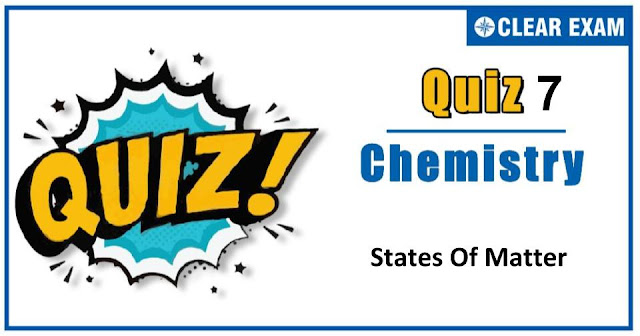## States of Matter Quiz-7

The one subject in NEET which is candidates who can easily attain good marks is Chemistry. That's the reason, often, one doesn’t pay notice and choose to compromise it. But if one wants to rank above others, the tip is to be thorough with NEET chemistry concepts. The understanding of reactions and definite basic understanding is what requires major attention in Chemistry but once done it only gets simpler from there. The main focus on the to-do list should be on getting a hang of the NCERT syllabus of NEET chemistry..

Q1. Frenkel defect is found in crystals in which the radius ration is
•  1.5
•  1.7
•  Very low
•  Slightly less than unity
Solution
(c) Frenkel defect is observed in the crystals in which the radius ratio is low

Q2.Avogadro’s number is the number of molecules present at NTP in:
•  1 mL of gas
•  1 litre of gas
•  22.4 litre of gas
•  22.4 mL of gas
Solution

Q3. In which one of the following does the given amount of chlorine exert the least pressure in a vessel of capacity 1 dm3 at 273 K?
•  0.0355g
•  0.071
•  6.023×1021 molecules
•  0.02 moles
Solution
(a) Ideal gas equation is Vp=nRT When V and T are same, p∝n Thus, when number of moles, i.e.,n is least, it will exert least pressure. (a) n=(wt.)/(mol.wt.)=0.0355/33.5=1×10-3 mol (b) n=0.071/33.5=2×10-3 mol (c) n=(number of molecules )/NA =(6.023×1021)/(6.023×1023 )=0.01 mol (d) n=0.02 mol Thus, 0.0335 g chlorine will exert the least pressure.

Q4. Which of the following statements is not true?
•  The ratio of the mean speed to the RMS speed is independent of the temperature
•  The square of the mean speed of the molecules is equal to the mean squared speed at a certain temperature
•  Mean kinetic energy of the gas molecules at any given temperature is independent of the mean speed
•  The difference between RMS speed and mean speed at any temperature for different gases diminishes as larger and yet larger molar masses are considered

•  p1>p2
•  p1<p2
•  p1=p2
•  All of these
Solution
(b) At constant pressure V∝T, but according to Gay Lussac’s law the pressure of a given mass of a gas is directly proportional to the absolute temperature. Thus, p1<p2

Q6. Oxygen gas is collected by downward displacement of water in a jar. The level of water inside the jar is adjusted to the height of water outside the jar. When the adjustment is made, the pressure exerted by the oxygen is:
•  Equal to the atmospheric pressure
•  Equal to the vapour pressure of oxygen at that temperature
•  Equal to atmospheric pressure plus aqueous tension at that temperature
•  Equal to atmospheric pressure minus aqueous tension at that temperature
Solution
(d) Pdry O2+Pwater vapour=Pwet O2

Q7.If the pressure of N2/H2 mixture in a closed vessel is 100 atmosphere and 20% of the mixture then reacts, the pressure at the same temperature would be:
•  The same
•  110 atmospheres
•  90 atmospheres
•  80 atmospheres
Solution
(c) 20% mixture produce 10% NH_3 N2+3H2→2NH3 Thus, percentage remains 90%

Q8.A certain gas takes three times as long to effuse out as helium. Its molecular mass will be:
•  27 u
•  36 u
•  64 u
•  9 u

Q9.Volume occupied by molecules of one mole gas at NTP, each having radius of 10-8 cm is:
•  22.0 litre
•  22.4 litre
•  10.9 mL
•  10.09 litre
Solution
(c) Volume of molecules in one mole =4×N×V=4×N×4/3 πr3 =4×6.023×1023×4/3×22/7×(10-8)3 =10.09 mL

Q10. At relatively high pressure, van der Waals’ equation reduces to:
•  PV=RT
•  PV=RT+a/V
•  PV=RT+Pb
•  PV=RT-a/V2
Solution
(c) At high pressure, volume of molecules should not be neglected in comparison to volume of gas. Also experimental studies reveals PV>RT at high P.#### Written by: AUTHORNAME

AUTHORDESCRIPTION## Want to know more

Please fill in the details below:

## Latest NEET Articles\$type=three\$c=3\$author=hide\$comment=hide\$rm=hide\$date=hide\$snippet=hide

Name

ltr
item
BEST NEET COACHING CENTER | BEST IIT JEE COACHING INSTITUTE | BEST NEET & IIT JEE COACHING: states-of-matter-quiz-7
states-of-matter-quiz-7
https://1.bp.blogspot.com/-ysV5klONbtY/X5gqMHmwggI/AAAAAAAAF98/JWeKh4rBNa8WvIBwtmvMTIReKRWLd27UgCLcBGAsYHQ/w640-h336/Quiz%2BImage%2B20%2B%25286%2529.jpg
https://1.bp.blogspot.com/-ysV5klONbtY/X5gqMHmwggI/AAAAAAAAF98/JWeKh4rBNa8WvIBwtmvMTIReKRWLd27UgCLcBGAsYHQ/s72-w640-c-h336/Quiz%2BImage%2B20%2B%25286%2529.jpg
BEST NEET COACHING CENTER | BEST IIT JEE COACHING INSTITUTE | BEST NEET & IIT JEE COACHING
https://www.cleariitmedical.com/2020/10/states-of-matter-quiz-7.html
https://www.cleariitmedical.com/
https://www.cleariitmedical.com/
https://www.cleariitmedical.com/2020/10/states-of-matter-quiz-7.html
true
7783647550433378923
UTF-8

STAY CONNECTED